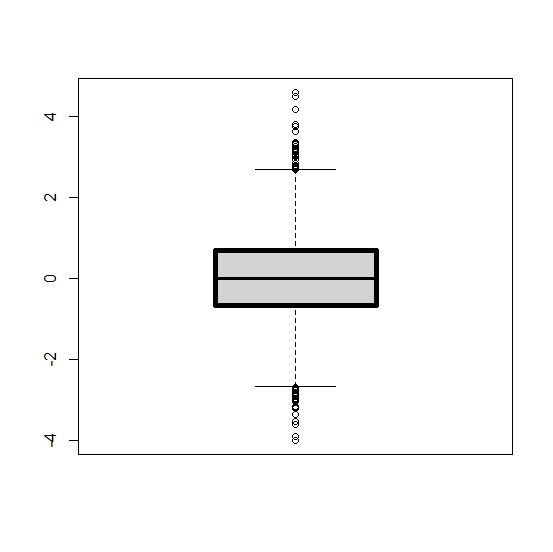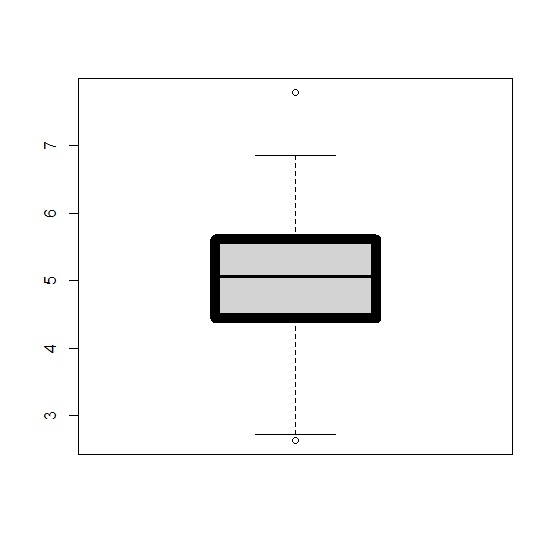# How to create boxplot in base R with higher width of the box lines?

To create boxplot in base R with higher width of the box lines, we can use the boxlwd argument inside boxplot function. For example, if we have a vector called x then we can create the boxplot with higher width of the box lines using the command −

boxplot(x,boxlwd=5)

## Example

Live Demo

x<-rnorm(10000)
boxplot(x,boxlwd=5)

## Output## Example

Live Demo

y<-rnorm(100,5,1)
boxplot(y,boxlwd=10)

## Output